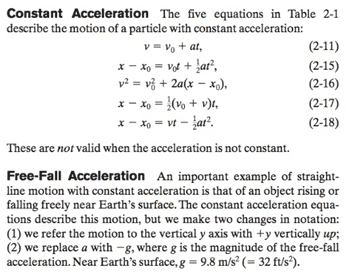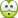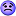# Ball dropped down from 5m, what is its speed when it hits the ground

• MrGoATi
In your problem, the direction of the initial velocity of the jump is not given. So, you actually don't know the initial x and y components of the velocity that you would plug into the constant-acceleration kinematics equations. By using the energy approach, you do not need to know the direction of the initial...

## Homework Statement

sportsman jumps at 5m/s velocity from 5m cliff to water. at what speed does he reach water?
so
given answer is 11.1m/s though in this book there are some wrong answers
(I did try other equations and I got right answer but I want to know why I can't get it using this one)
##g=-10m/s^2##
##v_0=-5m/s##
##h_0=0m##
h=-5m

## Homework Equations

I'm trying to do this using ##E_k1 + E_p1 = E_k2 + E_p2##
##E_k=\frac {mv^2}{2}##
##E_p=mgh=\frac {2mgh}{2}##

## The Attempt at a Solution

since ##h_0=0## ##E_p1=0##

putting in values

##\frac {mv_0^2}{2}=\frac {mv^2}{2}+\frac {2mgh}{2}##

after multiplying everything by 2 and dividing by m
##v_0^2=v^2+2gh##

##v^2=v_0^2-2gh##

##h=h_1-h_0##
##v^2=(-5)^2-2*(-10)*(-5-0)=25-100=-75##
v=root from -75=impossible. and root from 75 is wrong answer.

if I used one positive number in -2gh part I will get right answer. I think I might need to use |h| in these kind of formulas?

Last edited:
MrGoATi said:

## Homework Statement

sportsman jumps at 5m/s velocity from 5m cliff to water{/b}. at what speed does he reach water?
so
given answer is 11.1m/s though in this book there are some wrong answers
(I did try other equations and I got right answer but I want to know why I can't get it using this one)
##g=-10m/s^2##
##v_0=-5m/s##
##h_0=0m##
h=-5m

## Homework Equations

I'm trying to do this using ##E_k1 + E_p1 = E_k2 + E_p2##
##E_k=\frac {mv^2}{2}##
##E_p=mgh=\frac {2mgh}{2}##

## The Attempt at a Solution

since ##h_0=0## ##E_p1=0##

putting in values

##\frac {mv_0^2}{2}=\frac {mv^2}{2}+\frac {2mgh}{2}##

after multiplying everything by 2 and dividing by m
##v_0^2=v^2+2gh##

##v^2=v_0^2-2gh##

##h=h_1-h_0##
##v^2=(-5)^2-2*(-10)*(-5-0)=25-100=-75##
v=root from -75=impossible. and root from 75 is wrong answer.

if I used one positive number in -2gh part I will get right answer. I think I might need to use |h| in these kind of formulas?[/B]
It depends on how the person jumps from the top of the cliff. Do they jump straight out, or up some, or down some, etc.?

berkeman said:
It depends on how the person jumps from the top of the cliff. Do they jump straight out, or up some, or down some, etc.?

i guess it's as if the person is on a cliff and the cliff suddenly disappears and he is hit at the top of his head which gives him extra 5m/s velocity.

i got an answer on another post though, in ##E_p=mgh##
##g=-10m/s^2##

MrGoATi said:
i guess it's as if the person is on a cliff and the cliff suddenly disappears and he is hit at the top of his head which gives him extra 5m/s velocity.

Perhaps but that sounds a bit unlikely..

If someone jumps vertically off a cliff at 5m/s what is their velocity when they pass the cliff edge on the way back down?

•berkeman
MrGoATi said:
if I used one positive number in -2gh part I will get right answer. I think I might need to use |h| in these kind of formulas?

Stick with the equation...

V2 = U2 + 2gh

Then if up is +ve you correctly used g = -9.8m/s/s but what about the sign of h ?

Last edited:
CWatters said:
Stick with the equation...

V2 = U2 + 2gh

Then if up is +ve you correctly used g = -9.8m/s/s but what about the sign of h ?
sign of h is negative since down is always negative, and the travel was from higher altitude. g is usually negative but in this case it's somehow positive I got this question answered in another post, tho I'm not sure how I got multiple posts of this even though I made 1 XD.
TSny said:
In the potential energy formula mgh, g represents the magnitude of the acceleration of gravity. So, g is a positive quantity.

You defined your starting height as 0m, but that means you start at zero potential. This doesn't work and is the source of your negative. You should define your starting height as 5m and your ending height as 0m, when you have no more potential. Think about the potential (and more specifically the height) this way, if you're going down it's high to low, and if you're going up it's low to high.

MrGoATi said:
g is usually negative but in this case it's somehow positive
In my experience, very few textbooks ever use a negative value for the symbol ##g##. Here's an excerpt from Fundamentals of Physics by Halliday, et. al.With this convention, the magnitude of weight (in a free body diagram, say) is written simply as ##mg## rather than ##m|g|##. Of course, you are free to make your own sign conventions as long as your choice is clear to those who read your work.

In your problem, the direction of the initial velocity of the jump is not given. So, you actually don't know the initial x and y components of the velocity that you would plug into the constant-acceleration kinematics equations. By using the energy approach, you do not need to know the direction of the initial velocity.

•CWatters
If you start at 0m, define up as positive and assume the initial velocity was 5m/s upwards you get...

V2 = (+5)2 + 2(-9.8)(-5)

You get the same answer if you assume the initial velocity was 5m/s downwards.

CWatters said:
You get the same answer if you assume the initial velocity was 5m/s downwards.
You also get the same answer if you assume the initial velocity was 5 m/s outwards, split the initial velocity into x and y components, compute the final y component after the fall and then recombine with the unchanged x component using pythagoras.

•CWatters
CWatters said:
If you start at 0m, define up as positive and assume the initial velocity was 5m/s upwards you get...

V2 = (+5)2 + 2(-9.8)(-5)

You get the same answer if you assume the initial velocity was 5m/s downwards.
how could getting right answer by altering values,formulas and known data be helpful? like yeah I can easily get correct answer by changing it to your suggested ideas but when I'm at exam and I do not think of that when trying to do exercise I will still get wrong answer or if I use your idea in some much more complicated issue and then I would possibly get wrong answers again.
i'm trying to do this with maths and physics, not cheats that do not help for my future problems.

MrGoATi said:
how could getting right answer by altering values,formulas and known data be helpful? like yeah I can easily get correct answer by changing it to your suggested ideas but when I'm at exam and I do not think of that when trying to do exercise I will still get wrong answer or if I use your idea in some much more complicated issue and then I would possibly get wrong answers again.
i'm trying to do this with maths and physics, not cheats that do not help for my future problems.
These are all correct approaches. None of them are cheats. They correspond to assuming an upward initial velocity, a downward initial velocity or (as in the suggestion I offered), a sideways initial velocity. The resulting impact speed turns out to be the same regardless.

That makes sense since the final speed based on a conservation of energy argument must be the same regardless of the launch angle.

There is often more than one way to analyze a problem. Regardless of what correct analysis you do, the result will be the same.

TSny said:
In my experience, very few textbooks ever use a negative value for the symbol ##g##.
.
but g is usually used as a vector and it turns out negative most of the time does it not?
did not think of this that way until nowin ##F= mg##
##E=mgh##
##s=at^2/2##
it's not a vector so always positive?
and in
##g=v-u/t##
it depends on vector direction?

you just made physics more complicated for me.

jbriggs444 said:
These are all correct approaches. None of them are cheats. They correspond to assuming an upward initial velocity, a downward initial velocity or (as in the suggestion I offered), a sideways initial velocity. The resulting impact speed turns out to be the same regardless.

That makes sense since the final speed based on a conservation of energy argument must be the same regardless of the launch angle.

There is often more than one way to analyze a problem. Regardless of what correct analysis you do, the result will be the same.

ok I guess it's just too complicated for me to understand, the change of up and down makes sense, but pytagoras WT*

MrGoATi said:
but g is usually used as a vector and it turns out negative most of the time does it not?
Vectors aren't positive or negative. Scalars can be positive or negative. You might occasionally see the notation ##\vec{g}## or g to represent a vector that has magnitude 9.8 m/s2 (on earth) and direction downward. But, the symbol g (no arrow, no bold type) is conventionally used by virtually all the standard textbooks to denote the magnitude of the acceleration due to gravity. With this convention, g is a positive number.

If you choose the positive y direction to be upward, then in projectile motion the acceleration due to gravity would be in the negative y direction. So, with the usual convention for "g", you would write ay = -g. Thus, with g a positive number, ay is negative.

Also, this convention for "g" means that when a mass is at a height h above the ground, then it has gravitational potential energy relative to the ground of PE = mgh. Here, again, g is a positive number.

If you want to go against convention and define g to be negative; i.e., g = -9.8 m/s2, then you can do that as long as it is clear to those who read your work and as long as you write your equations correctly with this convention. For example, for a mass at a height h above the ground, you would now have to write the potential energy with a negative sign: PE = -mgh. PE would still come out positive, as before, but you can see that the signs are inconvenient here.

in ##F= mg##
##E=mgh##
it's not a vector so always positive?
Yes, that's right assuming the usual convention for the meaning of "g" as a positive number.
##s=at^2/2##
I'm not sure in what context you are using this equation. I don't see "g" here.
and in
##g=v-u/t##
it depends on vector direction?
I think you might have meant ##g = (v-u)/t##. I'm not sure how you are using this equation.

you just made physics more complicated for me.
Sorry, I'm trying to do the opposite.

Last edited:
MrGoATi said:
how could getting right answer by altering values,formulas and known data be helpful? like yeah I can easily get correct answer by changing it to your suggested ideas but when I'm at exam and I do not think of that when trying to do exercise I will still get wrong answer or if I use your idea in some much more complicated issue and then I would possibly get wrong answers again.
i'm trying to do this with maths and physics, not cheats that do not help for my future problems.

As far as I can see I didn't change anything. I used..

MrGoATi said:
g=−10m/s2g=−10m/s2g=-10m/s^2
v0=−5m/sv0=−5m/sv_0=-5m/s
h0=0mh0=0mh_0=0m
h=-5m

Edit: Ah ok so I used -9.8 instead of -10 for g. I missed that bit.

MrGoATi said:
ok I guess it's just too complicated for me to understand, the change of up and down makes sense, but pytagoras WT*

That wasn't Pythagoras ! that was the SUVAT equation of motion V2 = U2 + 2as with a=g=-9.8 and s=h=-5m.